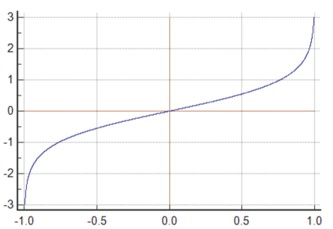# How to find the Hyperbolic Arc Tangent of a given value in Golang?

In this tutorial, we will learn how to find the Hyperbolic Arc Tangent of a given value in the Golang programming Language. Golang language has many packages with predefined functions that the developer can use without writing the complete logic.

To perform the mathematical operations and logic we have a math package in Golang. We will use this package only to find the Hyperbolic Arc Tangent of a given value. We will also see how to import the package and also how to call a function this package consists of by writing a Golang code.

## Hyperbolic Arc Tangent

### Definition

Hyperbolic Arc Tangent is a function similar to trigonometry functions. Hyperbolic Arc Tangent is equal to the analogs of the trigonometric function. The formula for Hyperbolic Arc Tangent is written below and the value of Hyperbolic Arc Tangent is at different angles.

### Syntax

atanh(x) = 1/2 * ln(1+x/1-x)


### Graph## Value of Hyperbolic Arc Tangent at different angles

• atanh(0) = 0

• atanh(30) = NaN

• atanh(45) = NaN

• atanh(60) = NaN

• atanh(90) = NaN

### Algorithm

Step 1 - Declaring the variable to store the value of the guardian and answer of float32 type.

Step 2 - Initializing the variable of a value.

Step 3 - Call the function of the Hyperbolic Arc Tangent and pass the value.

Step 4- Printing the result.

### Example

In this example, we will write a Golang program in which we will import a math package and call the Hyperbolic Arc Tangent function.

package main
import (

// fmt package provides the function to print anything
"fmt"

// math package provides multiple functions for different

// mathematical operations
"math"
)
func main() {

// declaring the variables to store the value of \value and answer
fmt.Println("Program to find the Hyperbolic Arc Tangent of a given value in the Golang programming language using a math package.")

// initializing the value of the variable value
value = 0.5

// finding Hyperbolic Arc Tangent for the given value

// printing the result
fmt.Println("The Hyperbolic Arc Tangent value with the value of", value, "is", answer)
}


### Output

Program to find the Hyperbolic Arc Tangent of a given value in the Golang programming language using a math package.
The Hyperbolic Arc Tangent value with the value of 0.5 is 0.5493061443340548


### Algorithm

Step 1 - Declaring the variable to store the value of the guardian and answer of float32 type.

Step 2 - Initializing the variable of a value.

Step 3 - Call the function of Hyperbolic Arc Tangent defined by us and pass the value as a parameter.

Step 4 - Printing the result.

### Example

In this example, we will write a Golang program in which we will import a math package and call the Hyperbolic Arc Tangent function in a separate function and call that function main.

package main
import
(
"fmt"
"math"
)
type of float64
func HyperbolicArcTangent(angle float64) float64 {

// returning the Hyperbolic Arc Tangent of the angle
return math.Atanh(angle)
}
func main() {

// declaring the variables to store the value of value and answer
fmt.Println("Program to find the Hyperbolic Arc Tangent of a given value in the Golang programming language using a separate function in the same program.")

// initializing the value of the value
value = 0.5

// finding Hyperbolic Arc Tangent for the given value in a separate function

// printing the result
fmt.Println("The Hyperbolic Arc Tangent value with the value of", value, "is", answer)
}


### Output

Program to find the Hyperbolic Arc Tangent of a given value in the Golang programming language using a separate function in the same program.
The Hyperbolic Arc Tangent value with the value of 0.5 is 0.5493061443340548


## Conclusion

These are two ways to find the Hyperbolic Arc Tangent by using the function in the math package and passing the value as a parameter. The second approach will provide abstraction in the program. To learn more about Golang you can explore these tutorials.